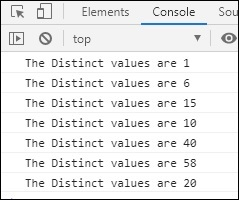# RxJS - Filtering Operator distinct

This operator will give all the values from the source observable that are distinct when compared with the previous value.

## Syntax

```distinct()
```

## Return value

It returns an observable that has distinct values.

## Example

```import { of } from 'rxjs';
import { distinct } from 'rxjs/operators';

let all_nums = of(1, 6, 15, 1, 10, 6, 40, 10, 58, 20, 40);
let final_val = all_nums.pipe(distinct());
final_val.subscribe(x => console.log("The Distinct values are "+x));
```

## Output